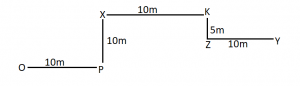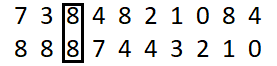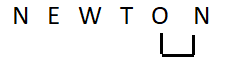Latest Banking jobs   »   Reasoning Ability Quiz For IBPS RRB...

# Reasoning Ability Quiz For IBPS RRB PO, Clerk Prelims 2021- 5th August

Directions (1-5): Study the following information carefully and answer the questions given below:
Eight persons i.e. P, Q, R, S, A, B, C and D are sitting at the corner of two square tables which one inside the other. Four persons are sitting at the corner of outer table and are facing towards the center of table while rest are sitting around the corner of inner table and are facing away from the center of table. P faces the one who sits to the immediate right of D. S does not sit next to P but sits on the same table on which P sits. B sits to the immediate left of C. B does not face P. One person sits between R and Q. Q does not face D. R faces towards the centre of table.

Q1. Four of the following five are alike in a certain way and hence they form a group. Which one of the following does not belong to that group?
(a) P-S
(b) P-A
(c) R-D
(d) S-C
(e) Q-B

Q2. Who among the following faces C?
(a) A
(b) B
(c) S
(d) P
(e) None of these

Q3. The number of persons sits between P and R, when counted from the left of P, is same as the number of persons sits between B and ___, when counted from the right of ___?
(a) Q
(b) A
(c) C
(d) S
(e) None of these

Q4. How many persons sits between A and C?
(a) two
(b) one
(c) three
(d) four
(e) None of these

Q5. Who among the following sits to the immediate left of A?
(a) P
(b) Q
(c) R
(d) B
(e) None of these

Directions (6-8): Study the following information carefully and answer the questions given below:
Point P is 10m to the East of Point O. Point P is 10m to the South of Point X. Point K is 10m to the East of Point X. Point K is 5m to the North of Point Z. Point Z is 10m to the West of Point Y.

Q6. In which direction is Point Y with respect to Point O?
(a) North West
(b) North East
(c) East
(d) South
(e) Cannot be determined

Q7. In which direction is Point Z with respect to Point X?
(a) North West
(b) North
(c) East
(d) South East
(e) Cannot be determined

Q8. In which direction is point X with respect to point Y?
(a) North West
(b) North
(c) East
(d) South
(e) Cannot be determined

Q9. How many such numerals are there in the number ‘7384821084’ which will remain at the Same position when arranged in ascending order from right to left?
(a) one
(b) two
(c) three
(d) four
(e) None

Q10. How many pairs of letters are there in the word ‘Newton’, each of which have as many letters between then in the word as they have between them in the English alphabet?
(a) one
(b) two
(c) three
(d) four
(e) None

Direction (11-15): In each of the questions below are given some statements followed by two conclusions. You have to take the given statements to be true even if they seem to be at variance with commonly known facts. Read all the conclusions and then decide which of the given conclusions logically follows from the given statements disregarding commonly known facts.
(a) If only conclusion I follows.
(b) If only conclusion II follows.
(c) If either conclusion I or II follows.
(d) If neither conclusion I nor II follows.
(e) If both conclusions I and II follow.

Q11. Statements: J>K>L; L<N<A; A=B
Conclusion I: J>A II: B>K

Q12. Statements: P>Q>R≥S<T=U<V
Conclusion I: V≥P II: P>S

Q13. Statements: J>K<L≤M<O=P>Q
Conclusion I: J≥M II: Q>K

Q14. Statements: Z>X=U; U<K<L; L>A
Conclusion I: L>X II: Z≤A

Q15. Statements: Q>R<S; S=T<V; V<N
Conclusion I: Q=N II: N>Q

Practice More Questions of Reasoning for Competitive Exams:

###### Target IBPS RRB PO and Clerk Prelims 2021

Solutions

Direction (1-5):
Sol.S1. Ans. (a)
S2. Ans. (c)
S3. Ans. (c)
S4. Ans. (b)
S5. Ans. (e)

Direction (6-8):S6. Ans. (b)
S7. Ans. (d)
S8. Ans. (a)

S9. Ans. (a)
Sol.S10. Ans. (a)
Sol.S11. Ans. (d)
Sol. I: J>A (False) II: B>K (False)

S12. Ans. (b)
Sol. I: V≥P (False) II: P>S (True)

S13. Ans. (d)
Sol. I: J≥M (False) II: Q>K (False)

S14. Ans. (a)
Sol. I: L>X (True) II: Z≤A (False)

S15. Ans. (d)
Sol. I: Q=N (False) II: N>Q (False)#### Congratulations!Union Budget 2023-24: Free PDF